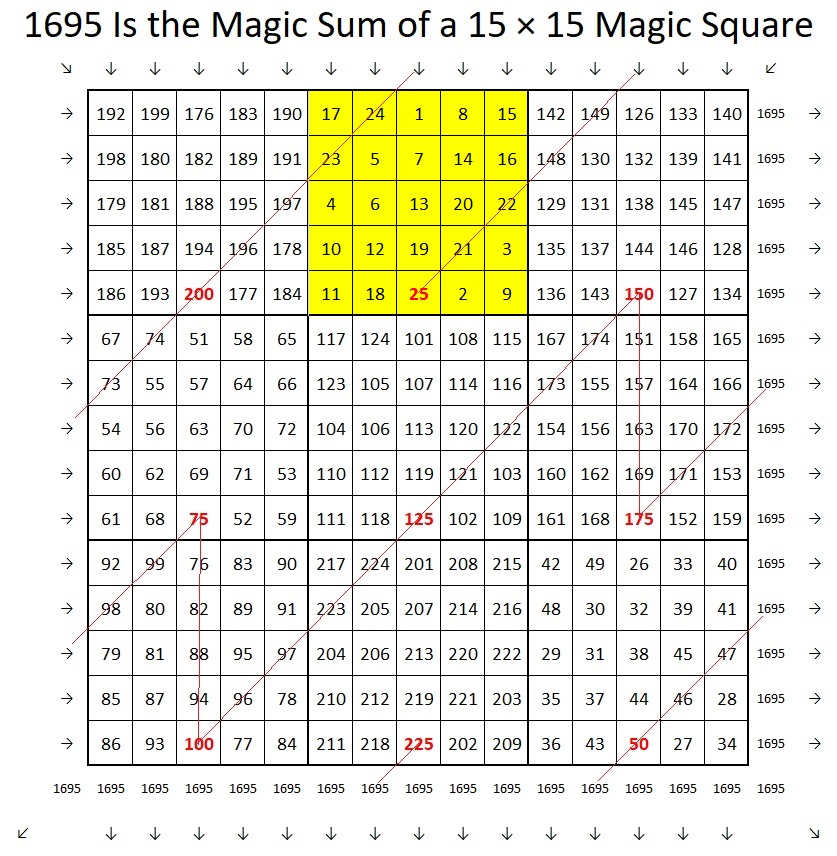# 1695 If You Can Solve 3×3 and 5×5 Magic Squares, Then You Can Solve a 15×15 Magic Square!

Contents

### Today’s Puzzle:

Completing a 15 × 15 magic square may seem daunting, but I assure you, if you can solve a 3 × 3 magic square and a 5 × 5 magic square, then you can complete a 15 × 15 magic square!

The 15 × 15 magic square below is made with twenty-five 3 × 3 magic squares. See the most famous 3 × 3 square in yellow? Do you see that the first 25 multiples of 9 are along the diagonals I’ve drawn? Do you understand the pattern that was used to make this magic square? Study it, then without looking at this one, can you make your own? Open this Excel file, 12 Factors 1683-1695, enable editing, and the sums of each row, column, and diagonal will automatically populate as you type in the numbers.This next 15 × 15 magic square is made with nine 5 × 5 magic squares. The one in yellow is one of MANY possible 5 × 5 squares. Do you see the first nine multiples of 25 along the diagonals I’ve drawn? Once you understand this pattern, perhaps you would like to take a turn duplicating it. Try it yourself on that same Excel file, 12 Factors 1683-1695.### Factors of 1695:

• 1695 is a composite number.
• Prime factorization: 1695 = 3 × 5 × 113.
• 1695 has no exponents greater than 1 in its prime factorization, so √1695 cannot be simplified.
• The exponents in the prime factorization are 1, 1, and 1. Adding one to each exponent and multiplying we get (1 + 1)(1 + 1)(1 + 1) = 2 × 2 × 2 = 8. Therefore 1695 has exactly 8 factors.
• The factors of 1695 are outlined with their factor pair partners in the graphic below.### More About the Number 1695:

Since 1695 is the sum of three consecutive numbers, it is the magic sum of a particular 3 × 3 Magic Square. Those three consecutive numbers are in yellow in the square below.Also, since 1695 is the sum of the five consecutive numbers shown in yellow below, it is the magic sum of this 5 × 5 magic square:And lastly, 1695 is the sum of the 15 consecutive numbers shown in yellow below, so here is yet another 15 × 15 magic square with 1695 as the magic sum. This magic square uses the same pattern that works for all odd number magic squares. It is so satisfying to complete it yourself. Study this one and then give it a try! Open that same Excel file, 12 Factors 1683-1695, to make Excel to all the adding for you.1695 is also the hypotenuse in FOUR Pythagorean triples:
225-1680-1695, which is 15 times (15-112-113),
828-1479-1695, which is 3 times (276-493-565)
1017-1356-1695, which is (3-4-5) times 339, and
1188-1209-1695, which is 3 times (396-403-565).

Did you notice that 565, 339, or 113 was a center number in every magic square in this post?

I hope you enjoy completing these magic squares on your own as you explore the number 1695.

This site uses Akismet to reduce spam. Learn how your comment data is processed.